/

### ERM Glossary: Risk model

A (portfolio) risk model is defined in Market Consistency by M.H.D. Kemp as ‘a mathematical framework for estimating the future spread of returns / outcomes a portfolio might generate were its positions versus its benchmark to remain unaltered in the future’*.

More generally it is any formulation of how risks might arise that is amenable to mathematical analysis.

Multivariate normal risk models

A particularly simple mathematical risk model is one in which the behaviour of future returns / outcomes is characterised by a multivariate normal distribution with parameters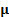(a vector of assumed returns for each asset) and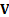(a covariance matrix).

Often in an asset management context each element ofwould be assumed to be the same constant value (i.e. we do not presume any ability to outperform the benchmark). Without loss of generality this constant can be set equal to zero if we are focusing on returns relative to a benchmark.

The easiest risk statistic that can be derived from such a model is the (ex-ante) tracking error,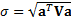where the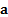correspond to the active positions versus the benchmark (i.e. each element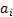ofis defined as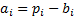, where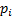is the weight of the’th security in the portfolio and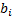is its weight in the benchmark).

For this model type, other risk statistics such as Value-at-Risk (VaR) for a given confidence level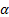can be derived from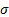by referring to the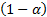quantile of a normal distribution with standard deviation. Such a VaR model is often called the variance-covariance VaR (particularly ifis based on the historical covariance matrix of different return series).

* Or, if the positions were to change in any prespecified dynamical manner under consideration